# [Neural Networks and Deep Learning] week3. Shallow Neural Network

[TOC]

## Neural Networks Overview

new notation:

• superscript `[i]` for quantities in layer i. (compared to superscript `(i)` for ith training example).
• subscript `i` for ith unit in a layer

## Neural Network Representation

notation:

• `a^[i]`: activation at layer i.
• input layer: x, layer 0.
• hidden layer
• output layer: prediction (yhat)
• don't count input layer as a layer

a 2 layer NN:## Computing a Neural Network's Output

each node in NN: 2 step computation

• z = wx + b
• a = sigmoid(z)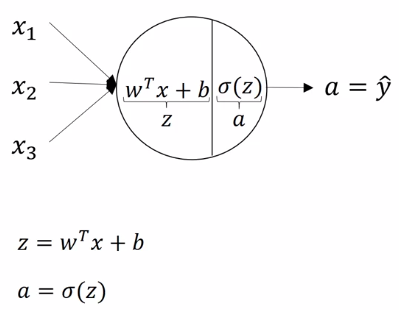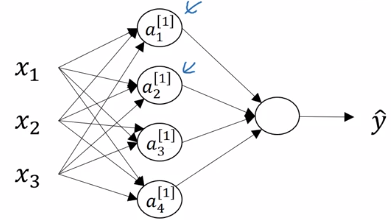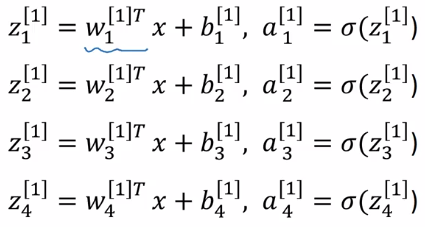`z^` = stacking `z_i`s vertically `a^` = sigmoid(`z^`) vectorize computing `z^`: W = stacking rows of wi.TW.shape = (4,3) b.shape = (4,1)

• input at layer i = `a^[i-1]` (`x = a`)
• output of each layer: `a[i] = sigmoid(W[i] a^[i-1] + b[i])`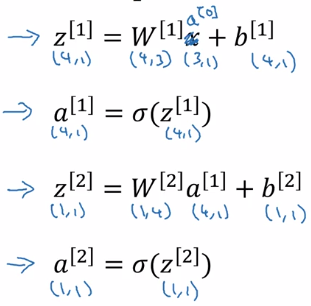## Vectorizing across multiple examples

vectorize the computation acrosse m examples. training examples: x^(1)...x^(m)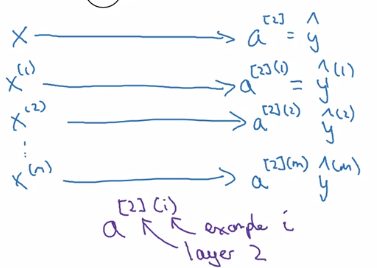computing all yhat(i) using forloop: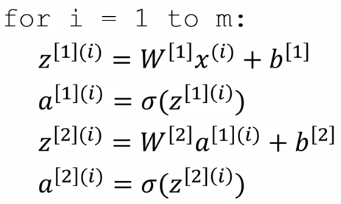X = stacking columns of x(i), `X = [x(1)...x(m)]` Z = stacking columns of z1 = [z1...z1] A = stacking columns of a(i)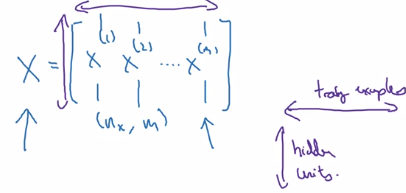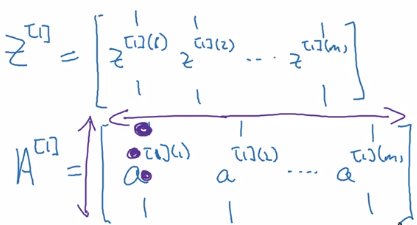horizontal index = training example `^(i)`
vertical index = nodes in layer `_i`/ input feature`x_i`

• Z = W * X + b
• A = sigmoid(Z)
• Z = W * A + b
• A = sigmoid(Z) = Yhat

## Explanation for Vectorized ImplementationRecap: stacking columns of training examples `x(i)` and activations `a[l](i)`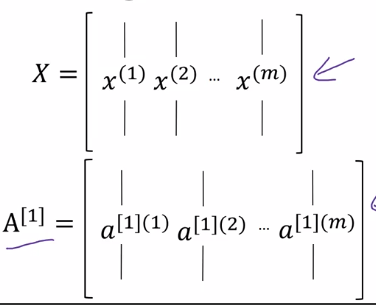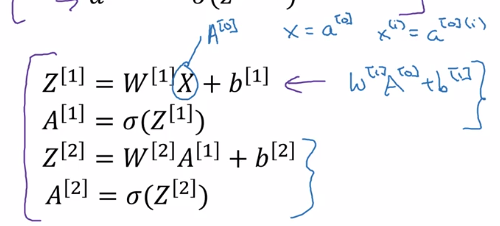## Activation functions

general case: `a = g(z)`, where g() is a nonlinear function.

• sigmoid: `a = 1 / (1 + exp(-z))`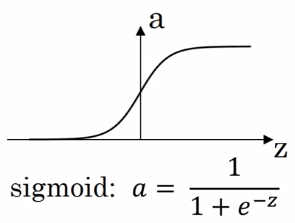a ∈ [0,1]

• tanh: `a = (exp(z) - exp(-z)) / (exp(z) + exp(-z))`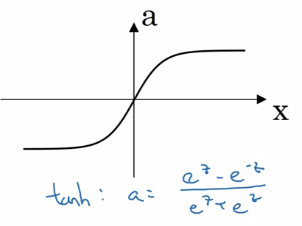a ∈ [-1, 1] — shifted sigmoid function ⇒ data is centered, learning for next layer easier almost always better than sigmoid, except for output layer (yhat = probability ∈[0,1])

downside of sigmoid and tanh: slope very small when |z| is large — GD slow. ⇒ ReLU

• ReLU `a = max(0, z)`

da/dz = 1 or 0 NN learns faster because slope is constant when |z| large disadvantage: da/dz = 0 when z<0 → leaky ReLU: small slope when z<0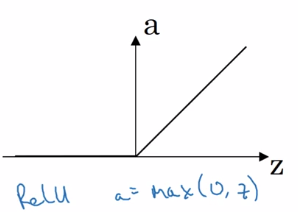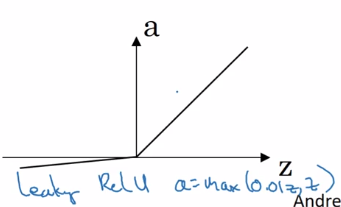Rules of thumb:

• output layer: sigmoid for binary classification (output probability), otherwise never use sigmoid
• hidden layer: use ReLU activation by default

## Why do you need non-linear activation functions?

use a linear activation function g(z) = z ? ⇒ `yhat` will just be a linear function of `x`. `yhat = Wx+b`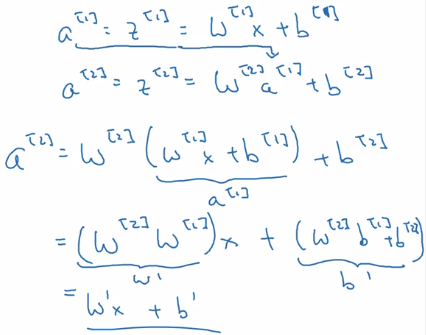one single place when using linear activation: in output layer ( y∈R )when doing regression

## Derivatives of activation functions

formulas for g'(z)

### g = sigmoid`g'(z) = g(z) * (1 - g(z)) = a * (1-a)`

• when z = +inf, g(z) = 1, g'(z) = 1*(1-1) = 0
• when z = -inf, g(z) = 0, g'(z) = 0
• when z = 0, g(z) = 0.5, g'(z) = 0.25

### g = tanh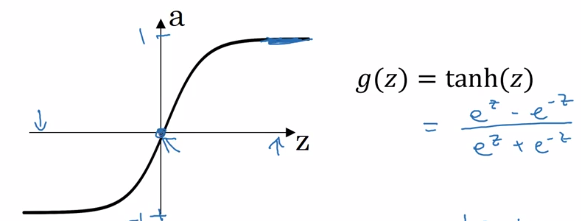`g'(z) = 1 - tanh(z)^2 = 1 - a^2`

• when z = +inf, tanh(z) = 1, g' = 0
• when z = -inf, tanh(z) = -1, g' = 0
• when z = 0, tanh(z) = 0, g' = 1

### g = ReLU / Leaky ReLU

ReLU: g(z) = max(0, z) g' is subgradient:

• g' = 0 when z<0
• g' = 1 when z>=0

Leaky ReLU: g(z) = max(0.01z, z)

• g' = 0.01 when z<0
• g' = 1 when z>=0

## Gradient descent for Neural Networks

NN with single hidden layer: n = nx, n = hidden layer size, n = 1 params: w, b, w, b

• `w.shape=(n, n), b.shape=(n, 1)`
• `w.shape=(n, n) , b.shape=(n,1)`
• output: yhat = a

cost function J(w,b,w,b) = 1/m * sum(L(yhat, y))

Gradient descent:

• random initialization
• repeat:
• compute dw, db, dw, db
• w := w - alpha*dw, ...

Fwd prop:general formular for `l`th layer: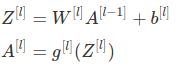Bck prop: computing derivatives `dw`, `db` note: use `keepdims = True` or `.rehape()` to avoid rank-1 arraies.## Backpropagation intuition (optional)

Derive the formulas using computation graph + chain rule.gradient for a single example `x=x(i), y=y(i)`: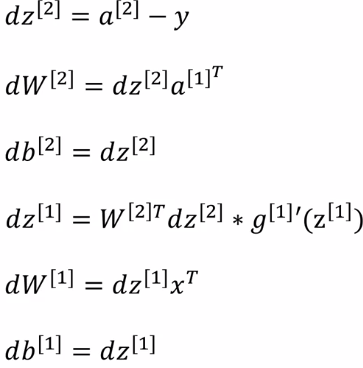vectorized implementation for i=1,..,m: stacking columns:`X = [x(1),..,x(m)]`, `Z = [z(1)...z(m)]`, `Y = [y(1)..y(m)]`, →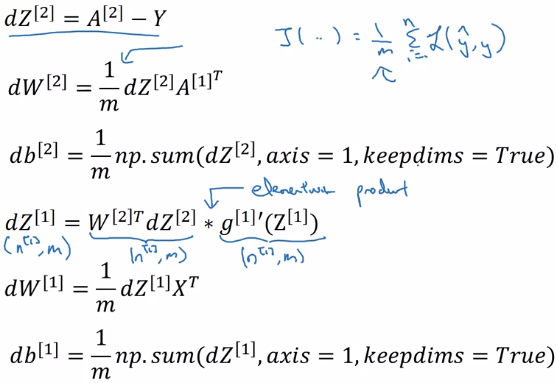## Random Initialization

Unlike logistic regression, needs init params randomly.

If we init all `w` and `b` to zeros: all activations `a_i` and `a_j` will be equal → `dz_i = dz_j`all hidden units completely identical ⇒ needs to init all params random, small number (small because we want have larger derivatives for sigmoid, which is at small values, to speed up gd). when w is init to small rand, b don't need random init.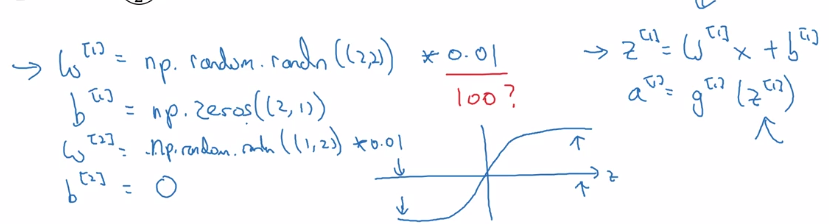#### Part 3 of series «Andrew Ng Deep Learning MOOC»：

comments powered by Disqus